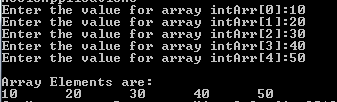Return Pointer from Function in C Programming

Above examples described how to create a pointer to function and how to use them in function. Suppose we have a simple function which accepts  the integer values and stores in the array. This array is then returned to the main function and is then displayed in the main function. This array is local to the function which accepts its elements. If the main function has to use the same array, then we have returned the pointer to the array to the main function.

In below program, intArr is declared inside the function fn_input. Its return value is a integer pointer (int*). The function accepts the input from the user and stores it in the array. Once all the values are accepted, it returns the pointer to the array itself. In the main program, integer pointer, intPtr is created and accepts the output of the function fn_input. This accepts the pointer to the array in the function. When array values are printed, it points to the memory location of the array in the function and displays the value present there.

#include <stdio.h>
#define ROWS 5

int* fn_input(){
static int   intArr[ROWS];
int i;

// Requests users to enter the value for elements
for (i = 0; i< ROWS; i++) {
printf("Enter the value for array intArr[%d]:", i);
scanf("%d", &intArr[i]);
}
return intArr; // return array pointer
}

int main()
{
int *intPtr;
int i;

intPtr = fn_input(); // accepts the pointer to an array

printf("\nArray Elements are:\n");
for (i = 0; i< ROWS; i++)
printf("%d\t", *(intPtr + i)); // displays the values as if it is accessing the array itself

return 0;
}